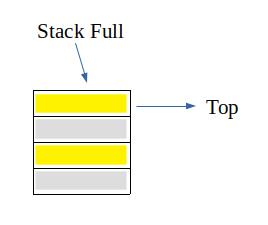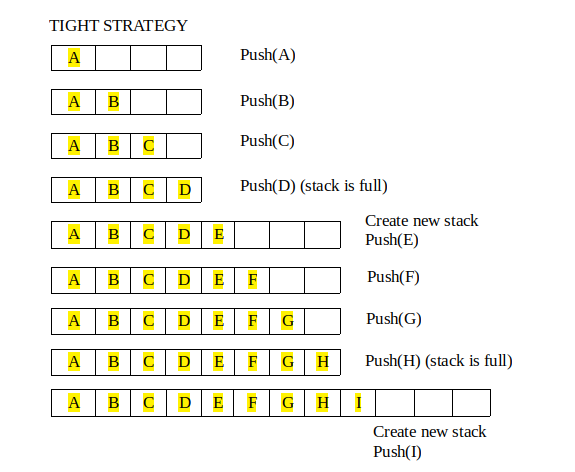# Growable array based stack

We all know about Stacks also known as Last-In-First-Out(LIFO) structures. Stack primarily has two main operation namely push and pop, where push inserts an element at top and pop removes an element from top of the stack.

Now, whenever an implementation of stack is considered its size is pre-determined or fixed. Even though it is dynamically allocated, still once it is made its size cannot be changed. And hence a condition called “stack full” arises.But what if a stack can grow as more elements are inserted or more elements are going to be inserted in future. Remember, we are talking about array based Stack. Growable Stack is the concept of allocating more memory such that “stack full” condition does not arises easily.

A Growable array-based Stack can be implemented by allocating new memory larger than previous stack memory and copying elements from old stack to new stack. And then at last change the name of new stack to the name which was given to old stack

There are two strategy for growable stack:
1. Tight Strategy : Add a constant amount to the old stack (N+c)
2. Growth Strategy : Double the size of old stack (2N)There are two operation on growable stack:
1. Regular Push Operation: Add one element at top of stack
2. Special Push Operation: Create a new stack of size greater than old stack (according to one of the strategy above) and copy all elements from old stack and then push the new element to the new stack.

## C++

 `// CPP Program to implement growable array based stack ` `// using tight strategy ` `#include ` `using` `namespace` `std; ` ` `  `// constant amount at which stack is increased ` `#define BOUND 4 ` ` `  `// top of the stack ` `int` `top = -1; ` ` `  `// length of stack ` `int` `length = 0; ` ` `  `// function to create new stack ` `int``* create_new(``int``* a) ` `{ ` `    ``// allocate memory for new stack ` `    ``int``* new_a = ``new` `int``[length + BOUND]; ` ` `  `    ``// copying the content of old stack ` `    ``for` `(``int` `i = 0; i < length; i++) ` `        ``new_a[i] = a[i]; ` ` `  `    ``// re-sizing the length ` `    ``length += BOUND; ` `    ``return` `new_a; ` `} ` ` `  `// function to push new element ` `int``* push(``int``* a, ``int` `element) ` `{ ` `    ``// if stack is full, create new one ` `    ``if` `(top == length - 1) ` `        ``a = create_new(a); ` ` `  `    ``// insert element at top of the stack ` `    ``a[++top] = element; ` `    ``return` `a; ` `} ` ` `  `// function to pop an element ` `void` `pop(``int``* a) ` `{ ` `    ``top--; ` `} ` ` `  `// function to display ` `void` `display(``int``* a) ` `{ ` `    ``// if top is -1, that means stack is empty ` `    ``if` `(top == -1) ` `        ``cout << ``"Stack is Empty"` `<< endl; ` `    ``else` `{ ` `        ``cout << ``"Stack: "``; ` `        ``for` `(``int` `i = 0; i <= top; i++) ` `            ``cout << a[i] << ``" "``; ` `        ``cout << endl; ` `    ``} ` `} ` ` `  `// Driver Code ` `int` `main() ` `{ ` `    ``// creating initial stack ` `    ``int` `*a = create_new(a); ` ` `  `    ``// pushing element to top of stack ` `    ``a = push(a, 1); ` `    ``a = push(a, 2); ` `    ``a = push(a, 3); ` `    ``a = push(a, 4); ` `    ``display(a); ` ` `  `    ``// pushing more element when stack is full ` `    ``a = push(a, 5); ` `    ``a = push(a, 6); ` `    ``display(a); ` ` `  `    ``a = push(a, 7); ` `    ``a = push(a, 8); ` `    ``display(a); ` ` `  `    ``// pushing more element so that stack can grow ` `    ``a = push(a, 9); ` `    ``display(a); ` ` `  `    ``return` `0; ` `} `

## Java

 `// Java Program to implement growable array based stack ` `// using tight strategy ` `class` `GFG  ` `{ ` ` `  `// constant amount at which stack is increased ` `static` `final` `int` `BOUND = ``4``; ` ` `  `// top of the stack ` `static` `int` `top = -``1``; ` ` `  `// length of stack ` `static` `int` `length = ``0``; ` ` `  `// function to create new stack ` `static` `int``[] create_new(``int``[] a) ` `{ ` `    ``// allocate memory for new stack ` `    ``int``[] new_a = ``new` `int``[length + BOUND]; ` ` `  `    ``// copying the content of old stack ` `    ``for` `(``int` `i = ``0``; i < length; i++) ` `        ``new_a[i] = a[i]; ` ` `  `    ``// re-sizing the length ` `    ``length += BOUND; ` `    ``return` `new_a; ` `} ` ` `  `// function to push new element ` `static` `int``[] push(``int``[] a, ``int` `element) ` `{ ` `    ``// if stack is full, create new one ` `    ``if` `(top == length - ``1``) ` `        ``a = create_new(a); ` ` `  `    ``// insert element at top of the stack ` `    ``a[++top] = element; ` `    ``return` `a; ` `} ` ` `  `// function to pop an element ` `static` `void` `pop(``int``[] a) ` `{ ` `    ``top--; ` `} ` ` `  `// function to display ` `static` `void` `display(``int``[] a) ` `{ ` `    ``// if top is -1, that means stack is empty ` `    ``if` `(top == -``1``) ` `        ``System.out.println(``"Stack is Empty"``); ` `    ``else`  `    ``{ ` `        ``System.out.print(``"Stack: "``); ` `        ``for` `(``int` `i = ``0``; i <= top; i++) ` `            ``System.out.print(a[i] + ``" "``); ` `        ``System.out.println(); ` `    ``} ` `} ` ` `  `// Driver Code ` `public` `static` `void` `main(String args[])  ` `{ ` `    ``// creating initial stack ` `    ``int` `[]a = create_new(``new` `int``[length + BOUND]); ` ` `  `    ``// pushing element to top of stack ` `    ``a = push(a, ``1``); ` `    ``a = push(a, ``2``); ` `    ``a = push(a, ``3``); ` `    ``a = push(a, ``4``); ` `    ``display(a); ` ` `  `    ``// pushing more element when stack is full ` `    ``a = push(a, ``5``); ` `    ``a = push(a, ``6``); ` `    ``display(a); ` ` `  `    ``a = push(a, ``7``); ` `    ``a = push(a, ``8``); ` `    ``display(a); ` ` `  `    ``// pushing more element so that stack can grow ` `    ``a = push(a, ``9``); ` `    ``display(a); ` `} ` `} ` ` `  `// This code is contributed by Princi Singh `

## C#

 `// C# Program to implement growable array based stack ` `// using tight strategy ` `using` `System;  ` `     `  `class` `GFG  ` `{ ` ` `  `// constant amount at which stack is increased ` `static` `int` `BOUND = 4; ` ` `  `// top of the stack ` `static` `int` `top = -1; ` ` `  `// length of stack ` `static` `int` `length = 0; ` ` `  `// function to create new stack ` `static` `int``[] create_new(``int``[] a) ` `{ ` `    ``// allocate memory for new stack ` `    ``int``[] new_a = ``new` `int``[length + BOUND]; ` ` `  `    ``// copying the content of old stack ` `    ``for` `(``int` `i = 0; i < length; i++) ` `        ``new_a[i] = a[i]; ` ` `  `    ``// re-sizing the length ` `    ``length += BOUND; ` `    ``return` `new_a; ` `} ` ` `  `// function to push new element ` `static` `int``[] push(``int``[] a, ``int` `element) ` `{ ` `    ``// if stack is full, create new one ` `    ``if` `(top == length - 1) ` `        ``a = create_new(a); ` ` `  `    ``// insert element at top of the stack ` `    ``a[++top] = element; ` `    ``return` `a; ` `} ` ` `  `// function to pop an element ` `static` `void` `pop(``int``[] a) ` `{ ` `    ``top--; ` `} ` ` `  `// function to display ` `static` `void` `display(``int``[] a) ` `{ ` `    ``// if top is -1, that means stack is empty ` `    ``if` `(top == -1) ` `        ``Console.WriteLine(``"Stack is Empty"``); ` `    ``else` `    ``{ ` `        ``Console.Write(``"Stack: "``); ` `        ``for` `(``int` `i = 0; i <= top; i++) ` `            ``Console.Write(a[i] + ``" "``); ` `        ``Console.WriteLine(); ` `    ``} ` `} ` ` `  `// Driver Code ` `public` `static` `void` `Main(String []args)  ` `{ ` `    ``// creating initial stack ` `    ``int` `[]a = create_new(``new` `int``[length + BOUND]); ` ` `  `    ``// pushing element to top of stack ` `    ``a = push(a, 1); ` `    ``a = push(a, 2); ` `    ``a = push(a, 3); ` `    ``a = push(a, 4); ` `    ``display(a); ` ` `  `    ``// pushing more element when stack is full ` `    ``a = push(a, 5); ` `    ``a = push(a, 6); ` `    ``display(a); ` ` `  `    ``a = push(a, 7); ` `    ``a = push(a, 8); ` `    ``display(a); ` ` `  `    ``// pushing more element so that stack can grow ` `    ``a = push(a, 9); ` `    ``display(a); ` `} ` `} ` ` `  `// This code is contributed by 29AjayKumar `

Output:

```Stack: 1 2 3 4
Stack: 1 2 3 4 5 6
Stack: 1 2 3 4 5 6 7 8
Stack: 1 2 3 4 5 6 7 8 9
```

Attention reader! Don’t stop learning now. Get hold of all the important DSA concepts with the DSA Self Paced Course at a student-friendly price and become industry ready.

My Personal Notes arrow_drop_upCheck out this Author's contributed articles.

If you like GeeksforGeeks and would like to contribute, you can also write an article using contribute.geeksforgeeks.org or mail your article to contribute@geeksforgeeks.org. See your article appearing on the GeeksforGeeks main page and help other Geeks.

Please Improve this article if you find anything incorrect by clicking on the "Improve Article" button below.

Improved By : princi singh, 29AjayKumar

Article Tags :
Practice Tags :

2

Please write to us at contribute@geeksforgeeks.org to report any issue with the above content.